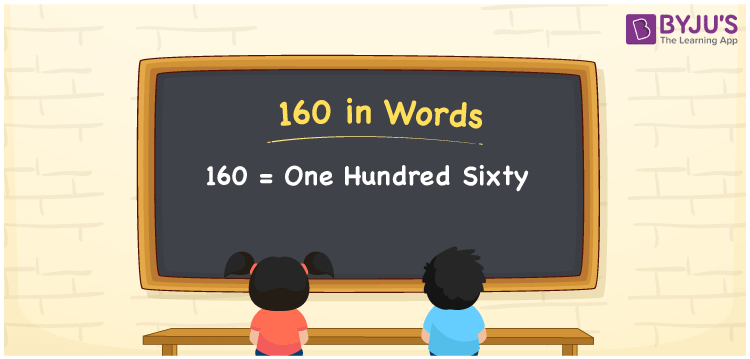# 160 in Words

160 in words is written as One hundred sixty. In both the International System of Numerals and the Indian System of Numerals, 160 is written as One hundred sixty. The number 160 is a Cardinal Number as it represents some quantity. For example, “Rita went to the market and spent 160 rupees”.

 160 in Words One hundred sixty One hundred sixty in Number 160

## 160 in English Words

We write 160 in English Words using the letters of the English alphabet. Therefore, we read 160 in English as “One hundred sixty.”## How to Write 160 in Words?

To write 160 in words, we shall use the place value chart. In the place value chart, write 1 in the hundreds, 6 in the tens, and 0 in the ones, respectively. Now let us make a place value chart to write the number 160 in words.

 Hundreds Tens Ones 1 6 0

Thus, we can write the expanded form as

1 × Hundred + 6 × Ten + 0 × One

= 1 × 100 + 6 × 10 + 0 × 1

= 100 + 60 + 0

= 160

= One hundred sixty.

160 is a natural number, the successor of 159 and the predecessor of 161.

160 in words – One hundred sixty

• Is 160 an odd number? – No
• Is 160 an even number? – Yes
• Is 160 a perfect square number? – No
• Is 160 a perfect cube number? – No
• Is 160 a prime number? – No
• Is 160 a composite number? – Yes

## Frequently Asked Questions on 160 in Words

Q1

### How to write 160 in words?

160 in words is written as One hundred sixty.
Q2

### How to write 160 in the International and Indian System of Numerals?

In both, the system of numerals, 160 in words, is written as One hundred sixty.
Q3

### What are the factors of 160?

The factors of 160 are 1, 2, 4, 5, 8, 10, 16, 20, 32, 40, 80 and 160.